## Hex Tutorial Puzzles

Tutorial Puzzle # 1

Start and solution pattern :Very simple - 9 moves.

Tutorial Puzzle # 2

Start and solution pattern :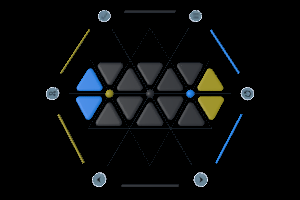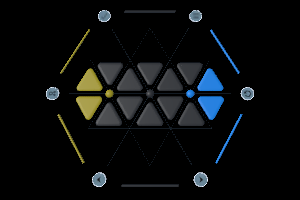Very simple - 15 moves.

Tutorial Puzzle # 3

Start and solution pattern :Very simple - 15 moves.

Tutorial Puzzle # 4

Start and solution pattern :Simple ?? moves.

Tutorial Puzzle # 5

Start and solution pattern :Simple ?? moves.

Tutorial Puzzle # 6

Start and solution pattern :Simple ?? moves.

Tutorial Puzzle # 7

Start and solution pattern :Simple ?? moves.

Tutorial Puzzle # 8

Start and solution pattern :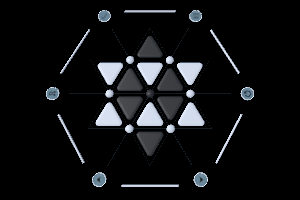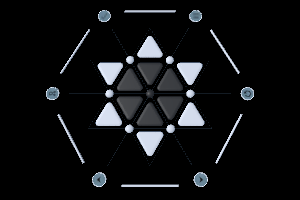Best solution found by ___________________ using  ???  moves.

Tutorial Puzzle # 9

Start and solution pattern :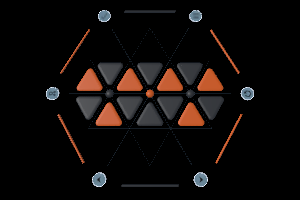Best solution found by ___________________ using  ???  moves.

The last two puzzles will be exchanged & the next two will be moved into the open section.

Tutorial Puzzle # 10

Start and solution pattern :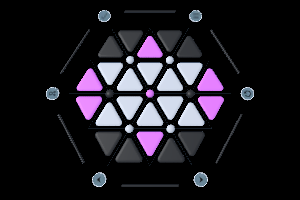Best solution found by ___________________ using  ???  moves.

Tutorial Puzzle # 11

Start and solution pattern :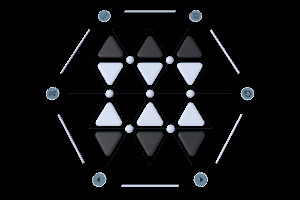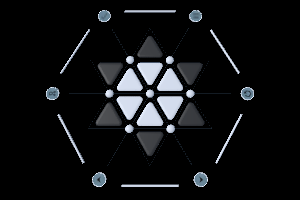Best solution found by ___________________ using  ???  moves.

Tutorial Puzzle # 12

Start and solution pattern :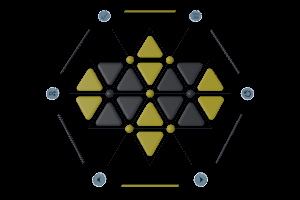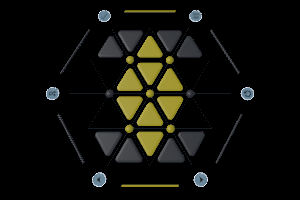Best solution found by ___________________ using  ???  moves.